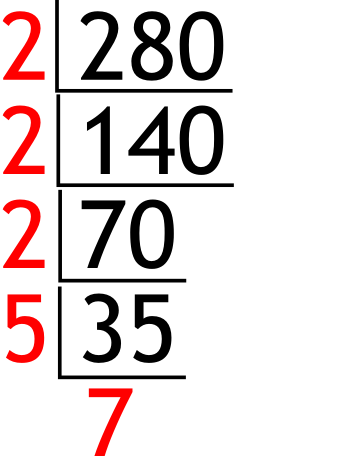The Singing Hedgehog Guide to:
Prime Factors 2 - Bookwork Method
Both 'proper' methods start with the lowest prime that divides into the value, and uses that until it won't go. Then we use the next prime that divides and so on.

Example:    Find the product of the prime factors of 2802 into 280 goes 140 and so on

35 is odd so doesn't divide by 2
3 does not divide exactly into 35 so we use 5

So the answer is 2x2x2x5x7 [or 23x5x7]

Next Method